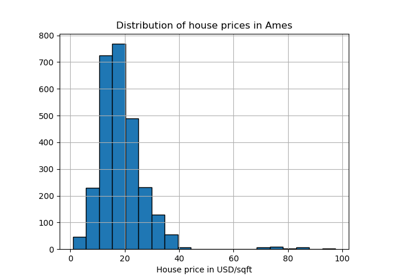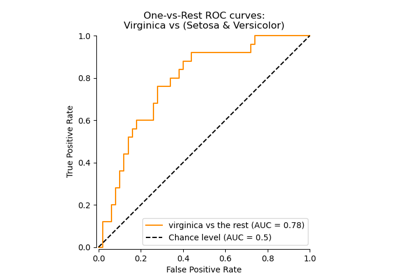# sklearn.preprocessing.LabelBinarizer¶

class sklearn.preprocessing.LabelBinarizer(*, neg_label=0, pos_label=1, sparse_output=False)[source]

Binarize labels in a one-vs-all fashion.

Several regression and binary classification algorithms are available in scikit-learn. A simple way to extend these algorithms to the multi-class classification case is to use the so-called one-vs-all scheme.

At learning time, this simply consists in learning one regressor or binary classifier per class. In doing so, one needs to convert multi-class labels to binary labels (belong or does not belong to the class). LabelBinarizer makes this process easy with the transform method.

At prediction time, one assigns the class for which the corresponding model gave the greatest confidence. LabelBinarizer makes this easy with the inverse_transform method.

Read more in the User Guide.

Parameters:
neg_labelint, default=0

Value with which negative labels must be encoded.

pos_labelint, default=1

Value with which positive labels must be encoded.

sparse_outputbool, default=False

True if the returned array from transform is desired to be in sparse CSR format.

Attributes:
classes_ndarray of shape (n_classes,)

Holds the label for each class.

y_type_str

Represents the type of the target data as evaluated by utils.multiclass.type_of_target. Possible type are ‘continuous’, ‘continuous-multioutput’, ‘binary’, ‘multiclass’, ‘multiclass-multioutput’, ‘multilabel-indicator’, and ‘unknown’.

sparse_input_bool

True if the input data to transform is given as a sparse matrix, False otherwise.

label_binarize

Function to perform the transform operation of LabelBinarizer with fixed classes.

OneHotEncoder

Encode categorical features using a one-hot aka one-of-K scheme.

Examples

>>> from sklearn import preprocessing
>>> lb = preprocessing.LabelBinarizer()
>>> lb.fit([1, 2, 6, 4, 2])
LabelBinarizer()
>>> lb.classes_
array([1, 2, 4, 6])
>>> lb.transform([1, 6])
array([[1, 0, 0, 0],
[0, 0, 0, 1]])


Binary targets transform to a column vector

>>> lb = preprocessing.LabelBinarizer()
>>> lb.fit_transform(['yes', 'no', 'no', 'yes'])
array([,
,
,
])


Passing a 2D matrix for multilabel classification

>>> import numpy as np
>>> lb.fit(np.array([[0, 1, 1], [1, 0, 0]]))
LabelBinarizer()
>>> lb.classes_
array([0, 1, 2])
>>> lb.transform([0, 1, 2, 1])
array([[1, 0, 0],
[0, 1, 0],
[0, 0, 1],
[0, 1, 0]])


Methods

 Fit label binarizer. Fit label binarizer/transform multi-class labels to binary labels. Get metadata routing of this object. get_params([deep]) Get parameters for this estimator. inverse_transform(Y[, threshold]) Transform binary labels back to multi-class labels. set_inverse_transform_request(*[, threshold]) Request metadata passed to the inverse_transform method. set_output(*[, transform]) Set output container. set_params(**params) Set the parameters of this estimator. Transform multi-class labels to binary labels.
fit(y)[source]

Fit label binarizer.

Parameters:
yndarray of shape (n_samples,) or (n_samples, n_classes)

Target values. The 2-d matrix should only contain 0 and 1, represents multilabel classification.

Returns:
selfobject

Returns the instance itself.

fit_transform(y)[source]

Fit label binarizer/transform multi-class labels to binary labels.

The output of transform is sometimes referred to as the 1-of-K coding scheme.

Parameters:
y{ndarray, sparse matrix} of shape (n_samples,) or (n_samples, n_classes)

Target values. The 2-d matrix should only contain 0 and 1, represents multilabel classification. Sparse matrix can be CSR, CSC, COO, DOK, or LIL.

Returns:
Y{ndarray, sparse matrix} of shape (n_samples, n_classes)

Shape will be (n_samples, 1) for binary problems. Sparse matrix will be of CSR format.

Get metadata routing of this object.

Please check User Guide on how the routing mechanism works.

Returns:

A MetadataRequest encapsulating routing information.

get_params(deep=True)[source]

Get parameters for this estimator.

Parameters:
deepbool, default=True

If True, will return the parameters for this estimator and contained subobjects that are estimators.

Returns:
paramsdict

Parameter names mapped to their values.

inverse_transform(Y, threshold=None)[source]

Transform binary labels back to multi-class labels.

Parameters:
Y{ndarray, sparse matrix} of shape (n_samples, n_classes)

Target values. All sparse matrices are converted to CSR before inverse transformation.

thresholdfloat, default=None

Threshold used in the binary and multi-label cases.

Use 0 when Y contains the output of decision_function (classifier). Use 0.5 when Y contains the output of predict_proba.

If None, the threshold is assumed to be half way between neg_label and pos_label.

Returns:
y{ndarray, sparse matrix} of shape (n_samples,)

Target values. Sparse matrix will be of CSR format.

Notes

In the case when the binary labels are fractional (probabilistic), inverse_transform chooses the class with the greatest value. Typically, this allows to use the output of a linear model’s decision_function method directly as the input of inverse_transform.

set_inverse_transform_request(*, threshold: Union[bool, None, str] = '$UNCHANGED$') [source]

Request metadata passed to the inverse_transform method.

Note that this method is only relevant if enable_metadata_routing=True (see sklearn.set_config). Please see User Guide on how the routing mechanism works.

The options for each parameter are:

• True: metadata is requested, and passed to inverse_transform if provided. The request is ignored if metadata is not provided.

• False: metadata is not requested and the meta-estimator will not pass it to inverse_transform.

• None: metadata is not requested, and the meta-estimator will raise an error if the user provides it.

• str: metadata should be passed to the meta-estimator with this given alias instead of the original name.

The default (sklearn.utils.metadata_routing.UNCHANGED) retains the existing request. This allows you to change the request for some parameters and not others.

New in version 1.3.

Note

This method is only relevant if this estimator is used as a sub-estimator of a meta-estimator, e.g. used inside a pipeline.Pipeline. Otherwise it has no effect.

Parameters:
thresholdstr, True, False, or None, default=sklearn.utils.metadata_routing.UNCHANGED

Metadata routing for threshold parameter in inverse_transform.

Returns:
selfobject

The updated object.

set_output(*, transform=None)[source]

Set output container.

See Introducing the set_output API for an example on how to use the API.

Parameters:
transform{“default”, “pandas”}, default=None

Configure output of transform and fit_transform.

• "default": Default output format of a transformer

• "pandas": DataFrame output

• None: Transform configuration is unchanged

Returns:
selfestimator instance

Estimator instance.

set_params(**params)[source]

Set the parameters of this estimator.

The method works on simple estimators as well as on nested objects (such as Pipeline). The latter have parameters of the form <component>__<parameter> so that it’s possible to update each component of a nested object.

Parameters:
**paramsdict

Estimator parameters.

Returns:
selfestimator instance

Estimator instance.

transform(y)[source]

Transform multi-class labels to binary labels.

The output of transform is sometimes referred to by some authors as the 1-of-K coding scheme.

Parameters:
y{array, sparse matrix} of shape (n_samples,) or (n_samples, n_classes)

Target values. The 2-d matrix should only contain 0 and 1, represents multilabel classification. Sparse matrix can be CSR, CSC, COO, DOK, or LIL.

Returns:
Y{ndarray, sparse matrix} of shape (n_samples, n_classes)

Shape will be (n_samples, 1) for binary problems. Sparse matrix will be of CSR format.

## Examples using sklearn.preprocessing.LabelBinarizer¶Evaluation of outlier detection estimators

Evaluation of outlier detection estimators